# F F T

### (Fast Fourier Transform)

Written by Paul Bourke
June 1993

Introduction

This document describes the Discrete Fourier Transform (DFT), that is, a Fourier Transform as applied to a discrete complex valued series. The mathematics will be given and source code (written in the C programming language) is provided in the appendices.

Theory

Continuous

For a continuous function of one variable f(t), the Fourier Transform F(f) will be defined as: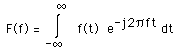and the inverse transform as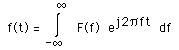where j is the square root of -1 and e denotes the natural exponentDiscrete

Consider a complex series x(k) with N samples of the form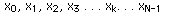where x is a complex number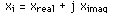Further, assume that that the series outside the range 0, N-1 is extended N-periodic, that is, xk = xk+N for all k. The FT of this series will be denoted X(k), it will also have N samples. The forward transform will be defined as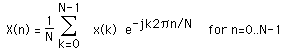The inverse transform will be defined as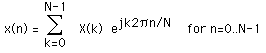Of course although the functions here are described as complex series, real valued series can be represented by setting the imaginary part to 0. In general, the transform into the frequency domain will be a complex valued function, that is, with magnitude and phase.The following diagrams show the relationship between the series index and the frequency domain sample index. Note the functions here are only diagrammatic, in general they are both complex valued series.For example if the series represents a time sequence of length T then the following illustrates the values in the frequency domain.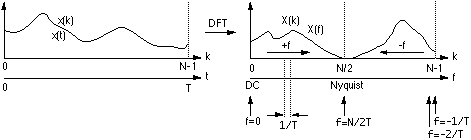Notes

• The first sample X(0) of the transformed series is the DC component, more commonly known as the average of the input series.

• The DFT of a real series, ie: imaginary part of x(k) = 0, results in a symmetric series about the Nyquist frequency. The negative frequency samples are also the inverse of the positive frequency samples.

• The highest positive (or negative) frequency sample is called the Nyquist frequency. This is the highest frequency component that should exist in the input series for the DFT to yield "uncorrupted" results. More specifically if there are no frequencies above Nyquist the original signal can be exactly reconstructed from the samples.

• The relationship between the harmonics returns by the DFT and the periodic component in the time domain is illustrated below.DFT and FFT algorithm.

While the DFT transform above can be applied to any complex valued series, in practice for large series it can take considerable time to compute, the time taken being proportional to the square of the number on points in the series. A much faster algorithm has been developed by Cooley and Tukey around 1965 called the FFT (Fast Fourier Transform). The only requirement of the the most popular implementation of this algorithm (Radix-2 Cooley-Tukey) is that the number of points in the series be a power of 2. The computing time for the radix-2 FFT is proportional to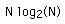So for example a transform on 1024 points using the DFT takes about 100 times longer than using the FFT, a significant speed increase. Note that in reality comparing speeds of various FFT routines is problematic, many of the reported timings have more to do with specific coding methods and their relationship to the hardware and operating system.

Sample transform pairs and relationships

• The Fourier transform is linear, that is

a f(t) + b g(t) ---> a F(f) + b G(f)

a xk + b yk ---> a Xk + b Yk

• Scaling relationship

f(t / a) ---> a F(a f)

f(a t) ---> F(f / a) / a

• Shifting

f(t + a) ---> F(f) e-j 2 pi a f

• Modulation

f(t) ej 2 pi a t ---> F(t - a)

• Duality

Xk ---> (1/N) xN-k

Applying the DFT twice results in a scaled, time reversed version of the original series.

• The transform of a constant function is a DC value only.• The transform of a delta function is a constant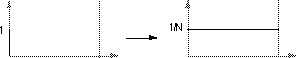• The transform of an infinite train of delta functions spaced by T is an infinite train of delta functions spaced by 1/T.• The transform of a cos function is a positive delta at the appropriate positive and negative frequency.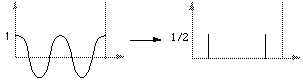• The transform of a sin function is a negative complex delta function at the appropriate positive frequency and a negative complex delta at the appropriate negative frequency.• The transform of a square pulse is a sinc function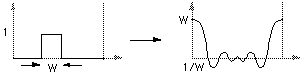More precisely, if f(t) = 1 for |t| < 0.5, and f(t) = 0 otherwise then F(f) = sin(pi f) / (pi f)

• Convolution

f(t) x g(t) ---> F(f) G(f)

F(f) x G(f) ---> f(t) g(t)

xk x yk ---> N Xk Yk

xk yk ---> (1/N) Xk x Yk

Multiplication in one domain is equivalent to convolution in the other domain and visa versa. For example the transform of a truncated sin function are two delta functions convolved with a sinc function, a truncated sin function is a sin function multiplied by a square pulse.

• The transform of a triangular pulse is a sinc2 function. This can be derived from first principles but is more easily derived by describing the triangular pulse as the convolution of two square pulses and using the convolution-multiplication relationship of the Fourier Transform.

Sampling theorem

The sampling theorem (often called "Shannons Sampling Theorem") states that a continuous signal must be discretely sampled at least twice the frequency of the highest frequency in the signal.

More precisely, a continuous function f(t) is completely defined by samples every 1/fs (fs is the sample frequency) if the frequency spectrum F(f) is zero for f > fs/2. fs/2 is called the Nyquist frequency and places the limit on the minimum sampling frequency when digitising a continuous signal.

If x(k) are the samples of f(t) every 1/fs then f(t) can be exactly reconstructed from these samples, if the sampling theorem has been satisfied, by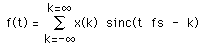where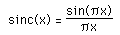Normally the signal to be digitised would be appropriately filtered before sampling to remove higher frequency components. If the sampling frequency is not high enough the high frequency components will wrap around and appear in other locations in the discrete spectrum, thus corrupting it.

The key features and consequences of sampling a continuous signal can be shown graphically as follows.

Consider a continuous signal in the time and frequency domain.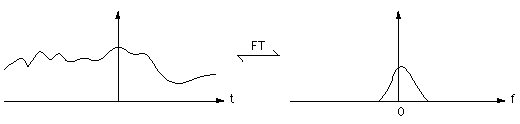Sample this signal with a sampling frequency fs, time between samples is 1/fs. This is equivalent to convolving in the frequency domain by delta function train with a spacing of fs.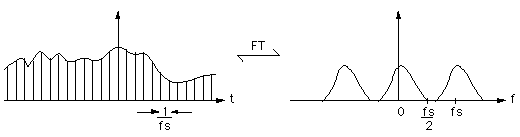If the sampling frequency is too low the frequency spectrum overlaps, and become corrupted.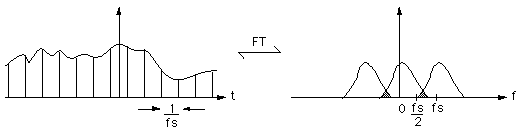Another way to look at this is to consider a sine function sampled twice per period (Nyquist rate). There are other sinusoid functions of higher frequencies that would give exactly the same samples and thus can't be distinguished from the frequency of the original sinusoid.

Appendix A. DFT (Discrete Fourier Transform)

```/*
Direct fourier transform
*/
int DFT(int dir,int m,double *x1,double *y1)
{
long i,k;
double arg;
double cosarg,sinarg;
double *x2=NULL,*y2=NULL;

x2 = malloc(m*sizeof(double));
y2 = malloc(m*sizeof(double));
if (x2 == NULL || y2 == NULL)
return(FALSE);

for (i=0;i<m;i++) {
x2[i] = 0;
y2[i] = 0;
arg = - dir * 2.0 * 3.141592654 * (double)i / (double)m;
for (k=0;k<m;k++) {
cosarg = cos(k * arg);
sinarg = sin(k * arg);
x2[i] += (x1[k] * cosarg - y1[k] * sinarg);
y2[i] += (x1[k] * sinarg + y1[k] * cosarg);
}
}

/* Copy the data back */
if (dir == 1) {
for (i=0;i<m;i++) {
x1[i] = x2[i] / (double)m;
y1[i] = y2[i] / (double)m;
}
} else {
for (i=0;i<m;i++) {
x1[i] = x2[i];
y1[i] = y2[i];
}
}

free(x2);
free(y2);
return(TRUE);
}```
Appendix B. FFT (Fast Fourier Transform)

```/*
This computes an in-place complex-to-complex FFT
x and y are the real and imaginary arrays of 2^m points.
dir =  1 gives forward transform
dir = -1 gives reverse transform
*/
short FFT(short int dir,long m,double *x,double *y)
{
long n,i,i1,j,k,i2,l,l1,l2;
double c1,c2,tx,ty,t1,t2,u1,u2,z;

/* Calculate the number of points */
n = 1;
for (i=0;i<m;i++)
n *= 2;

/* Do the bit reversal */
i2 = n >> 1;
j = 0;
for (i=0;i<n-1;i++) {
if (i < j) {
tx = x[i];
ty = y[i];
x[i] = x[j];
y[i] = y[j];
x[j] = tx;
y[j] = ty;
}
k = i2;
while (k <= j) {
j -= k;
k >>= 1;
}
j += k;
}

/* Compute the FFT */
c1 = -1.0;
c2 = 0.0;
l2 = 1;
for (l=0;l<m;l++) {
l1 = l2;
l2 <<= 1;
u1 = 1.0;
u2 = 0.0;
for (j=0;j<l1;j++) {
for (i=j;i<n;i+=l2) {
i1 = i + l1;
t1 = u1 * x[i1] - u2 * y[i1];
t2 = u1 * y[i1] + u2 * x[i1];
x[i1] = x[i] - t1;
y[i1] = y[i] - t2;
x[i] += t1;
y[i] += t2;
}
z =  u1 * c1 - u2 * c2;
u2 = u1 * c2 + u2 * c1;
u1 = z;
}
c2 = sqrt((1.0 - c1) / 2.0);
if (dir == 1)
c2 = -c2;
c1 = sqrt((1.0 + c1) / 2.0);
}

/* Scaling for forward transform */
if (dir == 1) {
for (i=0;i<n;i++) {
x[i] /= n;
y[i] /= n;
}
}

return(TRUE);
}
```
Modification by Peter Cusack that uses the MS complex type fft_ms.c.

References

Fast Fourier Transforms
Walker, J.S.
CRC Press. 1996

Fast Fourier Transforms: Algorithms
Elliot, D.F. and Rao, K.R.

Fast Fourier Transforms and Convolution Algorithms
Nussbaumer, H.J.
Springer, New York, 1982

Digital Signal Processing
Oppenheimer, A.V. and Shaffer, R.W.
Prentice-Hall, Englewood Cliffs, NJ, 1975

# 2 Dimensional FFT

Written by Paul Bourke
July 1998

The following briefly describes how to perform 2 dimensional Fourier transforms. Source code is given at the end and an example is presented where a simple low pass filtering is applied to an image. Filtering in the spatial frequency domain is advantageous for the same reasons as filtering in the frequency domain is used in time series analysis, the filtering is easier to apply and can be significantly faster.

It is assumed the reader is familiar with 1 dimensional Fourier transforms as well as the key time/frequency transform pairs.In the most general situation a 2 dimensional transform takes a complex array. The most common application is for image processing where each value in the array represents to a pixel, therefore the real value is the pixel value and the imaginary value is 0.

2 dimensional Fourier transforms simply involve a number of 1 dimensional Fourier transforms. More precisely, a 2 dimensional transform is achieved by first transforming each row, replacing each row with its transform and then transforming each column, replacing each column with its transform. Thus a 2D transform of a 1K by 1K image requires 2K 1D transforms. This follows directly from the definition of the Fourier transform of a continuous variable or the discrete Fourier transform of a discrete system.

The transform pairs that are commonly derived in 1 dimension can also be derived for the 2 dimensional situation. The 2 dimensional pairs can often be derived simply by considering the procedure of applying transforms to the rows and then the columns of the 2 dimensional array.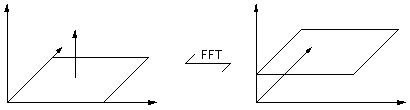Delta function transforms to a 2D DC plane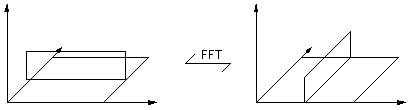Line of delta functions transforms to a line of delta functionsSquare pulse <--FFT-->2D sinc function

Note

The above example has had the quadrants reorganised so as to place DC (freq = 0) in the center of the image. The default organisation of the quadrants from most FFT routines is as below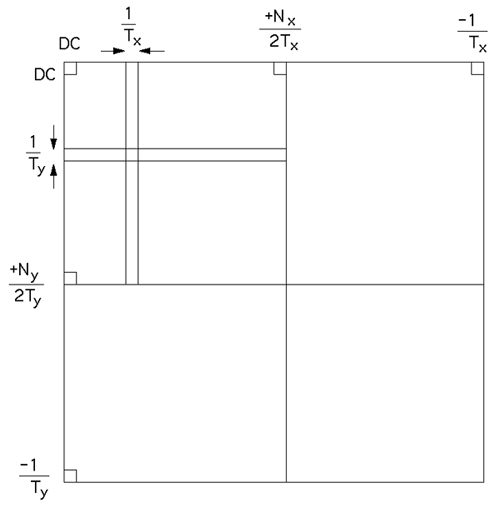### Example

The following example uses the image shown on the right.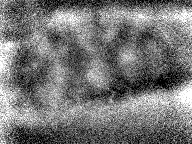In order to perform FFT (Fast Fourier Transform) instead of the much slower DFT (Discrete Fourier Transfer) the image must be transformed so that the width and height are an integer power of 2. This can be achieved in one of two ways, scale the image up to the nearest integer power of 2 or zero pad to the nearest integer power of 2. The second option was chosen here to facilitate comparisons with the original. The resulting image is 256 x 256 pixels.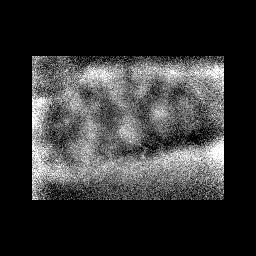The magnitude of the 2 dimension FFT (spatial frequency domain) is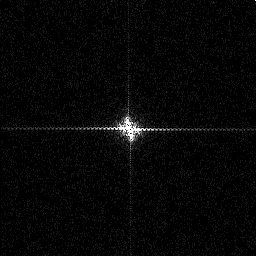Image processing can now be performed (for example filtering) and the image converted back to the spatial domain. For example low pass filtering involves reducing the high frequency components (those radially distant from the center of the above image). Two examples using different cut-off frequencies are illustrated below.Low pass filter with a low corner frequency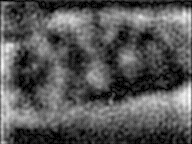Low pass filter with a higher corner frequency

### Source Code

```/*-------------------------------------------------------------------------
Perform a 2D FFT inplace given a complex 2D array
The direction dir, 1 for forward, -1 for reverse
The size of the array (nx,ny)
Return false if there are memory problems or
the dimensions are not powers of 2
*/
int FFT2D(COMPLEX **c,int nx,int ny,int dir)
{
int i,j;
int m,twopm;
double *real,*imag;

/* Transform the rows */
real = (double *)malloc(nx * sizeof(double));
imag = (double *)malloc(nx * sizeof(double));
if (real == NULL || imag == NULL)
return(FALSE);
if (!Powerof2(nx,&m,&twopm) || twopm != nx)
return(FALSE);
for (j=0;j<ny;j++) {
for (i=0;i<nx;i++) {
real[i] = c[i][j].real;
imag[i] = c[i][j].imag;
}
FFT(dir,m,real,imag);
for (i=0;i<nx;i++) {
c[i][j].real = real[i];
c[i][j].imag = imag[i];
}
}
free(real);
free(imag);

/* Transform the columns */
real = (double *)malloc(ny * sizeof(double));
imag = (double *)malloc(ny * sizeof(double));
if (real == NULL || imag == NULL)
return(FALSE);
if (!Powerof2(ny,&m,&twopm) || twopm != ny)
return(FALSE);
for (i=0;i<nx;i++) {
for (j=0;j<ny;j++) {
real[j] = c[i][j].real;
imag[j] = c[i][j].imag;
}
FFT(dir,m,real,imag);
for (j=0;j<ny;j++) {
c[i][j].real = real[j];
c[i][j].imag = imag[j];
}
}
free(real);
free(imag);

return(TRUE);
}

/*-------------------------------------------------------------------------
This computes an in-place complex-to-complex FFT
x and y are the real and imaginary arrays of 2^m points.
dir =  1 gives forward transform
dir = -1 gives reverse transform

Formula: forward
N-1
---
1   \          - j k 2 pi n / N
X(n) = ---   >   x(k) e                    = forward transform
N   /                                n=0..N-1
---
k=0

Formula: reverse
N-1
---
\          j k 2 pi n / N
X(n) =       >   x(k) e                    = forward transform
/                                n=0..N-1
---
k=0
*/
int FFT(int dir,int m,double *x,double *y)
{
long nn,i,i1,j,k,i2,l,l1,l2;
double c1,c2,tx,ty,t1,t2,u1,u2,z;

/* Calculate the number of points */
nn = 1;
for (i=0;i<m;i++)
nn *= 2;

/* Do the bit reversal */
i2 = nn >> 1;
j = 0;
for (i=0;i<nn-1;i++) {
if (i < j) {
tx = x[i];
ty = y[i];
x[i] = x[j];
y[i] = y[j];
x[j] = tx;
y[j] = ty;
}
k = i2;
while (k <= j) {
j -= k;
k >>= 1;
}
j += k;
}

/* Compute the FFT */
c1 = -1.0;
c2 = 0.0;
l2 = 1;
for (l=0;l<m;l++) {
l1 = l2;
l2 <<= 1;
u1 = 1.0;
u2 = 0.0;
for (j=0;j<l1;j++) {
for (i=j;i<nn;i+=l2) {
i1 = i + l1;
t1 = u1 * x[i1] - u2 * y[i1];
t2 = u1 * y[i1] + u2 * x[i1];
x[i1] = x[i] - t1;
y[i1] = y[i] - t2;
x[i] += t1;
y[i] += t2;
}
z =  u1 * c1 - u2 * c2;
u2 = u1 * c2 + u2 * c1;
u1 = z;
}
c2 = sqrt((1.0 - c1) / 2.0);
if (dir == 1)
c2 = -c2;
c1 = sqrt((1.0 + c1) / 2.0);
}

/* Scaling for forward transform */
if (dir == 1) {
for (i=0;i<nn;i++) {
x[i] /= (double)nn;
y[i] /= (double)nn;
}
}

return(TRUE);
}

/*-------------------------------------------------------------------------
Calculate the closest but lower power of two of a number
twopm = 2**m <= n
Return TRUE if 2**m == n
*/
int Powerof2(int n,int *m,int *twopm)
{
if (n <= 1) {
*m = 0;
*twopm = 1;
return(FALSE);
}

*m = 1;
*twopm = 2;
do {
(*m)++;
(*twopm) *= 2;
} while (2*(*twopm) <= n);

if (*twopm != n)
return(FALSE);
else
return(TRUE);
}

```